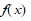# NMath User's Guide

Chapter 13. Calculus (.NET, C#, CSharp, VB, Visual Basic, F#)

NMath provides classes for encapsulating functions of one variable,. Once constructed, function objects enable you to:

evaluate a function at a given x-value or vector of x-values;

integrate a function over a given interval;

compute the derivative of a function at a given x-value;

manipulate functions algebraically.

This chapter describes how to create and manipulate function objects.

Top

Top